Example Questions

A fiber-optic Michelson interferometer is a device that detects changes in optical paths. In a fiber-optic interferometer, a coherent light source (usually a laser) is sent through a beam splitter that splits the light along two paths. These beams are coupled into fiber optic cables that can be arranged and manipulated more freely than mirrors. The two beams are finally recombined by a second beam splitter and superimposed on a screen. If there is a phase difference between the two waves, interference fringes will be viewed on the screen.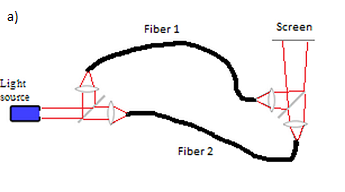By observing fringe shifts, one can quantify the change in optical path difference between the two fibers using the following equation: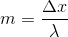where m is the number of fringe shifts, x is the difference between the optical paths of the two beams, and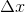is the change in the optical path difference.

Experiment 1:

A student sets up a fiber-optic Michelson interferometer and heats one of the fibers with various resistors and power supplies, fans air over one of the fibers, and then bends one of the fibers. The resulting fringe shifts, as well as the change in optical path difference (OPD), are shown below.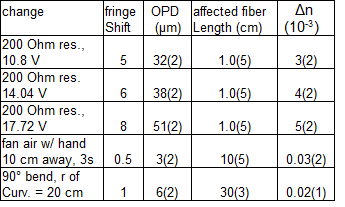Why might fiber-optic cables be used instead of mirrors to redirect the beams? Consider the following scenarios and determine which one would motivate the choice of fiber-optic cables rather than mirrors.

The interferometer must be used to measure changes in orbital distance between the moon and the Earth.

The interferometer must be used to measure the speed of sound.

The interferometer must be used in a miniature deformable device to detect temperature-induced expansion.

The interferometer must be used to measure the speed of light.

The interferometer must be used in a miniature deformable device to detect temperature-induced expansion.

Explanation:

The fiber-optic cables enable the optical paths to be easily arranged, which make them ideal for applications in smaller devices. Any experiment in which the interferometer is used to measure extremely long distances (e.g. speed of light, distance between two astronomical bodies) would be a poor use for fiber optics. Imagine the cost of a 100,000 mile fiber-optic cable!

A fiber-optic Michelson interferometer is a device that detects changes in optical paths. In a fiber-optic interferometer, a coherent light source (usually a laser) is sent through a beam splitter that splits the light along two paths. These beams are coupled into fiber optic cables that can be arranged and manipulated more freely than mirrors. The two beams are finally recombined by a second beam splitter and superimposed on a screen. If there is a phase difference between the two waves, interference fringes will be viewed on the screen.By observing fringe shifts, one can quantify the change in optical path difference between the two fibers using the following equation:where m is the number of fringe shifts, x is the difference between the optical paths of the two beams, andis the change in the optical path difference.

Experiment 1:

A student sets up a fiber-optic Michelson interferometer and heats one of the fibers with various resistors and power supplies, fans air over one of the fibers, and then bends one of the fibers. The resulting fringe shifts, as well as the change in optical path difference (OPD), are shown below.Why is it necessary to use a second beam splitter in this setup?

The first beam splitter reduces the intensity of the light, which must be compensated for.

The second beam splitter acts as a screen on which interference fringes can be observed.

The beams must be refocused since they are exiting from different points.

The beams must be recombined so that interference fringes are observed.

The beams must be recombined so that interference fringes are observed.

Explanation:

The second beam splitter recombines two beams. The interference fringes are only visible if the two beams are superimposed, as the question statement describes.

A scientist is exploring the nature of energy and work in two experimental systems.

Experiment 1

She first sets up a system with a ball on an inclined plane, and calculates the potential and kinetic energies of the ball at three positions. She places the ball at position 1, stops the ball after rolling to position 2, and then again allows it to roll to position 3. The measured kinetic and potential energies are shown in the table, along with measurements of the force of friction acting on the ball.

 Ball Position Potential Energy Kinetic Energy Force of Friction 1 150 kJ X kJ 0 N 2 75 kJ 75 kJ 0 N 3 X kJ 150 kJ 0 N

Experiment 2

The same scientist then sets up another experiment with the same ball, again at three positions.  The ball is provided a slight push, and then allowed to roll down three levels without any additional external input of energy.

She uses the following formulae to calculate the energy levels of the ball:

Potential Energy = Mass of the Ball x Acceleration Due to Gravity x Height of the Ball

Kinetic Energy = (1/2) x Mass of the Ball x (Velocity of the Ball)2

She also measures the internal energy of the ball, a value that she defines as the amount of energy contained in the motion of the molecules that make up the matter of the ball.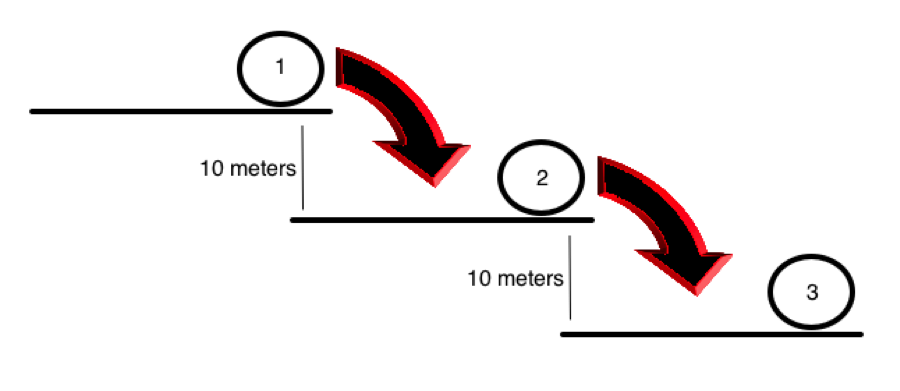Position Potential Energy Velocity Internal Energy 1 A 1 m/s 5 kJ 2 B 5.47 m/s 6 kJ 3 C 10.94 m/s 7 kJ

A second scientist is examining this data and wants to test if introducing an electric field around the ramp in Experiment 1 changes the measured energy values. To do this, he sets up another system, identical to that in Experiment 1, but with the addition of an electric field. He compares data generated by both the original system in Experiment 1 and the new system with the electric field. Experiment 1 is now serving as what?

An experimental group

A confounder

A control group

A variable

A modifier

A control group

Explanation:

A control group serves as a comparison group to an experimental group. The control group and the experimental group should be identical except in the presence of one variable that is being studied. In this case, that one variable is the presence of an electric field.

Sound waves travel through a medium by mechanically disturbing the particles of that medium. As particles in the medium are displaced by the sound wave, they in turn act upon neighboring particles. In this fashion, the wave travels through the medium through a parallel series of disturbed particles. Like in other forms of motion, the rate at which the sound wave travels can be measured by dividing the distance over which the wave travels by the time required for it to do so.

Study 1
A group of students hypothesizes that the velocity of sound is dependent upon the density of the medium through which it passes. They propose that with more matter in a given space, each particle needs to travel a shorter distance to disturb the adjacent particles. Using two microphones and a high speed recording device, the students measured the delay from the first microphone to the second. They chose a variety of media, shown in Table 1, and measured the velocity of sound through each using their two-microphone setup. The results are found in Table 1.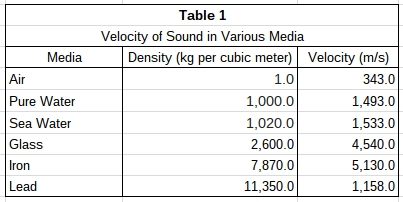Study 2
The students wanted to test their hypothesis by using the same medium at different densities. To do this, they heated pure water to various temperatures and repeated the procedure described in Study 1. Their results can be found in Table 2.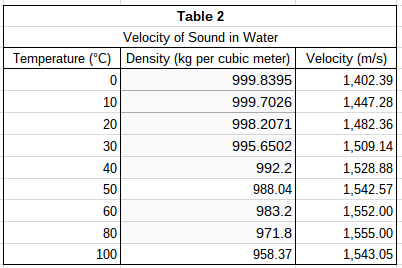Assume that density of a substance is the only contributing factor to velocity of sound through that substance. If the students' hypothesis in Study 1 is correct, what might they have predicted for the velocity of sound through lead? (Assume all other values in Table 1 remained the same.)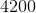m/s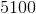m/s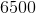m/sm/s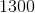m/sm/s

Explanation:

The students hypothesized that velocity of sound through a substance is directly dependent upon the density of that substance. In other words, as density increases, velocity of sound also increases. Because lead is the densest substance in Table 1, only velocities greater than 5,130 m/s (the velocity through iron) would satisfy the hypothesized relationship between velocity and density.

Sound waves travel through a medium by mechanically disturbing the particles of that medium. As particles in the medium are displaced by the sound wave, they in turn act upon neighboring particles. In this fashion, the wave travels through the medium through a parallel series of disturbed particles. Like in other forms of motion, the rate at which the sound wave travels can be measured by dividing the distance over which the wave travels by the time required for it to do so.

Study 1
A group of students hypothesizes that the velocity of sound is dependent upon the density of the medium through which it passes. They propose that with more matter in a given space, each particle needs to travel a shorter distance to disturb the adjacent particles. Using two microphones and a high speed recording device, the students measured the delay from the first microphone to the second. They chose a variety of media, shown in Table 1, and measured the velocity of sound through each using their two-microphone setup. The results are found in Table 1.Study 2
The students wanted to test their hypothesis by using the same medium at different densities. To do this, they heated pure water to various temperatures and repeated the procedure described in Study 1. Their results can be found in Table 2.Jackie read about this experiment and decided to recreate Study 1. She found that each of the velocities she measured are higher than those in the original experiment. What is one possible explanation for this difference?

Jackie used substances at a different temperature than those used in the original experiment.

Jackie didn't measure the density of each substance.

Jackie used a shorter sample of each substance.

Jackie used lower-quality microphones.

Jackie used substances at a different temperature than those used in the original experiment.

Explanation:

As study 2 shows, changes in temperature are correlated to changes in velocity. If Jackie used substances at a different temperature than those used in the original experiment, she would find different velocities of sound for those substances.

A shorter sample of each substance would not affect sound velocity, as the distance traveled is accounted for in the equation for velocity. The act of measuring the density of each substance would not affect the velocity of sound.

A student is doing an experiment relating force and distance to work and work and time to power. She is given the following table:

 Force Distance Work Time 3 Newtons 1 meter 3 Joules 10 Seconds 4 Newtons 10 meters 40 Joules 20 Seconds 1.5 Newtons 7 meters 10.5 Joules 1 Second

She is also told that:

Power = Work/ Time

Work is in Joules and Time is in seconds. Power is measured in Watts.

If the Watts of power equals the amount needed to light the light bulb attached then the bulb will be lit. If more Watts of power are present, an attached light bulb will burn brighter.

If the student were to do an experiemt with a force of 6 Newtons and a distance of 1 meter, how much work would the student be doing?

6 Joules

5.5 Joules

12 Joules

0.3 Watts

6 Joules

Explanation:

The table values show that the Work is calculated by multiplying the force by the distance (in this case 6x1=6 Joules of power).

A student is doing an experiment relating force and distance to work and work and time to power. She is given the following table:

 Force Distance Work Time 3 Newtons 1 meter 3 Joules 10 Seconds 4 Newtons 10 meters 40 Joules 20 Seconds 1.5 Newtons 7 meters 10.5 Joules 1 Second

She is also told that:

Power = Work/ Time

Work is in Joules and time is in seconds. Power is measured in Watts.

If the Watts of power equals the amount needed to light the light bulb attached then the bulb will be lit. If more Watts of power are present, an attached light bulb will burn brighter.

What is a Joule equal to according to the table?

Newton/ Meter

Newton x Watt

Newton x Meter

Newton/ Watt

Newton x Meter

Explanation:

From the table you can determine that the Unit for Work is a Joule and that Work is calculated by multiplying the force by the distance travelled. Since force is in Newtons and distance is shown in meters, you can infer that the unit for force multiplied by the unit for distance equals the unit for Work (Newton x Meter)

A student is doing an experiment relating force and distance to work and work and time to power. She is given the following table:

 Force Distance Work Time 3 Newtons 1 meter 3 Joules 10 Seconds 4 Newtons 10 meters 40 Joules 20 Seconds 1.5 Newtons 7 meters 10.5 Joules 1 Second

She is also told that:

Power = Work/ Time

Work is in Joules and time is in seconds. Power is measured in Watts.

If the Watts of power equals the amount needed to light the light bulb attached then the bulb will be lit. If more Watts of power are present, an attached light bulb will burn brighter.

If the student is trying to light a 2 Watt Bulb, which of the following experimental scenarios would work?

60 Joules of Work in 30 seconds

All of these

40 Joules of Work in 20 seconds

2 Joules of Work in a second

All of these

Explanation:

The formula Power (in Watts) = Work / Time is given in the experiment above. Since the light bulb is 2 Watts, it needs that many Watts of power to light it. All of the answers divide out to be 2 Watts of Power and therefore would all be enough to light the light bulb.

A student is doing an experiment relating force and distance to work and work and time to power. She is given the following table:

 Force Distance Work Time 3 Newtons 1 meter 3 Joules 10 Seconds 4 Newtons 10 meters 40 Joules 20 Seconds 1.5 Newtons 7 meters 10.5 Joules 1 Second

She is also told that:

Power = Work/ Time

Work is in Joules and time is in seconds. Power is measured in Watts.

If the Watts of power equals the amount needed to light the light bulb attached then the bulb will be lit. If more Watts of power are present, an attached light bulb will burn brighter.

The student tried the experiment again, but this time she moved the object over a distance of 40 meters, but in a circle so she ended up in the same place. She thinks that the distance would be 0 meters because she didn't change locations, so she cannot light a bulb because no power would be created. Is this true?

Yes because the distance would be 0 meters and the Work done and Power would also be 0.

Yes because the light bulb cannot work if she moves in a circle.

No because the experiment never discussed the paths taken, only the distance moved.

No because work has nothing to do with how far she travelled, only the force she used.

No because the experiment never discussed the paths taken, only the distance moved.

Explanation:

The experiment never discusses paths because that does not effect how much work took place. If that were true that no work is done when you move in a circle, running on a track would be easier than running in a straight path, which is not true. The correct answer is the only one that correctly states that the student's theory is false and gives a logical answer because force AND distance are factors in the Work done, not just the force.

A student is doing an experiment relating force and distance to work and work and time to power. She is given the following table:

 Force Distance Work Time 3 Newtons 1 meter 3 Joules 10 Seconds 4 Newtons 10 meters 40 Joules 20 Seconds 1.5 Newtons 7 meters 10.5 Joules 1 Second

She is also told that:

Power = Work/ Time

Work is in Joules and time is in seconds. Power is measured in Watts.

If the Watts of power equals the amount needed to light the light bulb attached then the bulb will be lit. If more Watts of power are present, an attached light bulb will burn brighter.

The student repeats the experiment at home and finds out that the light bulb burns brighter if she pushes the object up an incline rather than a flat surface of the same distance in the same amount of time. What would explain this?

The student must be using less force to push the object up the incline.

The student must be using more force to push the object up the incline.

Watts have nothing to do with the distance travelled or force used.

The student must have miscalculated.